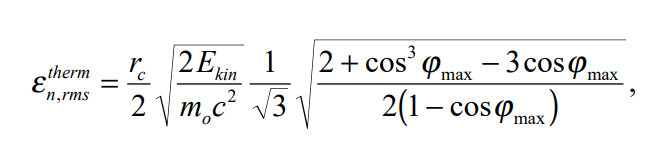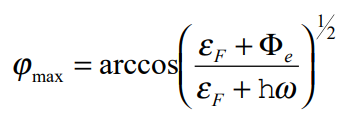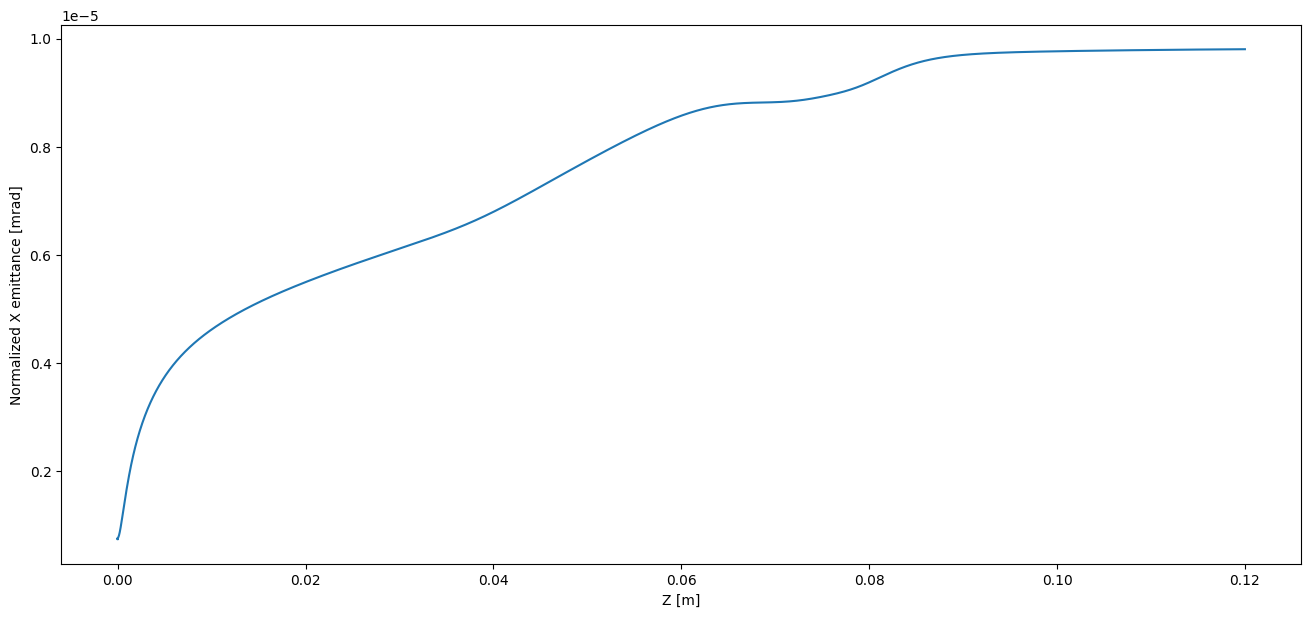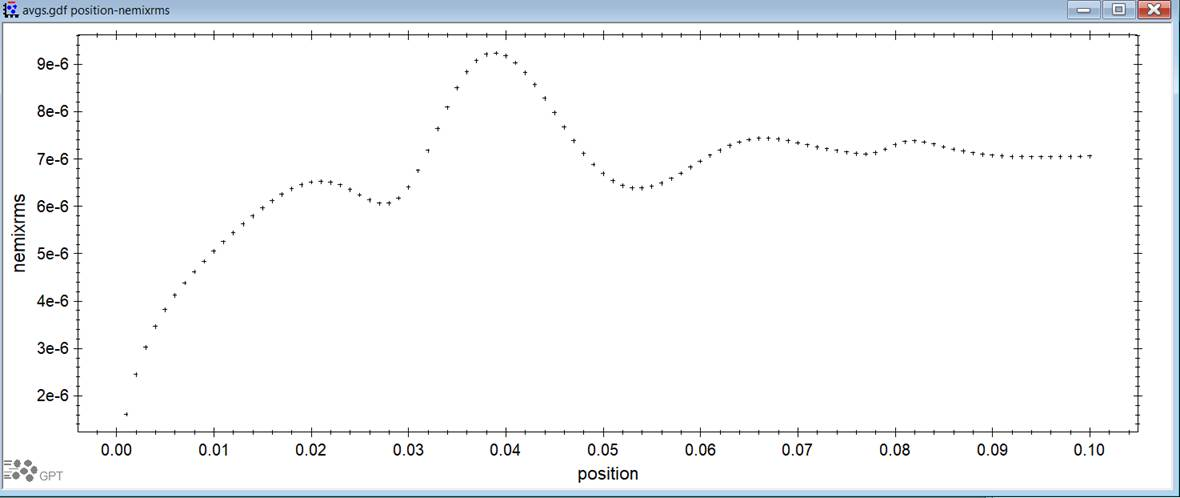# opal AT lists.psi.ch

Subject: The OPAL Discussion Forum

## Re: [Opal] Initial longitudinal momentum, thermal emittance, reference particle

• From: Zhi Li <zhili29882 AT gmail.com>
• To: "Adelmann Andreas (PSI)" <andreas.adelmann AT psi.ch>, opal AT lists.psi.ch, christof.j.kraus AT gmail.com
• Subject: Re: [Opal] Initial longitudinal momentum, thermal emittance, reference particle
• Date: Tue, 13 Dec 2022 14:07:05 -0600

Thank you very much for clarifying questions 1) and 3).

Now that I have done more searching, regarding the latter part of question 2), if I set my monitor position to be nanometers away from the cathode I should be expecting a captured value for emittance that is very close to a value calculated by the formula below, correct? NONQUIL model takes into account of Schotty effect right?4) Recently my colleague made a simulation with the same field map, same distribution parameters using GPT and I realized that the normalized x-emittance plot looks very different. To my understanding, there was a benchmark done by  N. R. Neveu et al. and the results seem to agree between the two codes. Can you help take a guess what might be causing this discrepancy? I can send the input file for the GPT simulation if you need it. Thanks again for the help!

Best regards,

ZhiOn Tue, Dec 6, 2022 at 3:05 PM Adelmann Andreas (PSI) <andreas.adelmann AT psi.ch> wrote:
Hi Zhi, great that you are using OPAL!

Questions:

1) If I generate my initial distribution using the NONEQUIL emission model, does the longitudinal momentum of the particles from this override the longitudinal momentum (P0) define in the BEAM parameters? If yes, how do I set this BEAM parameter longitudinal momentum (P0) to 0?

Yes P0 is overwritten. In case you have problems setting P0=0.0, then try P0=1.0E-12.

2) Is there a way to have the thermal emittance value saved to the output file (.stat) from the emitted distribution by NONEQUIL? Perhaps I can define a monitor very close to the cathode and capture this value? So far the closest I can set the monitor to the cathode is one micrometer away, is there a way to set it to 0 so its right ontop of the cathode?

Yes with a monitor you can achieve this, however a distance of 0.0 will not work. The minimal distance is determined by the time step, the smaller
the time step the closer you can put the monitor towards the cathode.

What is the exact formula that OPAL uses to calculate this thermal emittance? I want to confirm the thermal emittance value captured by a monitor with theoretical calculation by plugging in laser energy, work function, cathode temp, and Fermi energy into an equation.

I think I do not understand the question. In case of a monitor you have access to the phase space and can calculate the emittance “by hand”. Other
than that the emission process is described in chapter 15.4 of the manual (http://amas.web.psi.ch/opal/Documentation/2022.1/#sec.distribution.emissionmodel ).

3) In the output (.stat) file, I can plot the field seen by the reference particle. What is the exact definition of this reference particle? I don't seem to find a good description of this in the user manual. Is this the first particle emitted from the cathode or does it represent the average of every particle in the bunch?

If you plot against column 2, it uses the path length (m) in the floor coordinate system of the on-axis particle if I remember correctly.

Cheer Andy

Best regards,

Zhi

Here is my input file:

OPTION, PSDUMPFREQ = 300;
OPTION, STATDUMPFREQ = 1;
OPTION, BOUNDPDESTROYFQ=10;
OPTION, AUTOPHASE=4;

OPTION, VERSION = 20300;

Title, string="Original AWA Photoinjector input file modified";

//----------------------------------------------------------------------------
//Global Parameters

REAL rf_freq             = 2.998e3;     //RF frequency. (MHz)
REAL n_particles         = 1E3;      //Number of particles in simulation.
REAL beam_bunch_charge   = 1e-3;      //Charge of bunch. (C)

//Initial Momentum Calculation
REAL Edes    = 1e-9; //initial energy in GeV
REAL gamma   = (Edes+EMASS)/EMASS;
REAL beta    = sqrt(1-(1/gamma^2));
REAL P0      = gamma*beta*EMASS;    //inital z momentum

//Printing initial energy and momentum to terminal output.
value , {Edes, P0};

//----------------------------------------------------------------------------
REAL gun_inj_phase = 0;

GUN:    RFCavity, L = 0.1, VOLT = 110.26, ELEMEDGE = 0.0, TYPE = "STANDING",
FMAPFN = "DriveGunGPTmap.T7", FREQ = 2998, LAG = (gun_inj_phase*Pi)/180.0,
APVETO = TRUE;
//----------------------------------------------------------------------------
// DEFINE BEAM LINE

myLine:  Line = (GUN);
//M: MONITOR, ELEMEDGE = 1e-9, OUTFN = 'mon';

// Complete accelerator
DRIVE: Line = (myLine);
//----------------------------------------------------------------------------
Dist:DISTRIBUTION, TYPE = GAUSS,
SIGMAR = 0.001,
CUTOFFR = 2.0,
SIGMAT = 1e-12,
CUTOFFLONG = 2.0,
NBIN = 10,
EMISSIONSTEPS = 100,
EMISSIONMODEL = NONEQUIL,
ELASER = 6.48,
W = 4.31,
FE = 7.0,
CATHTEMP = 300,
EMITTED = TRUE,
WRITETOFILE = True;
//----------------------------------------------------------------------------
FS_SC: Fieldsolver, FSTYPE = FFT,
MX = 10, MY = 10, MT = 10,
PARFFTX = True,
PARFFTY = True,
PARFFTT = True,
BCFFTX = open,
BCFFTY = open,
BCFFTT = open,
BBOXINCR = 1,
GREENSF = INTEGRATED;
//----------------------------------------------------------------------------
// Electron Beam Definition

BEAM1:  BEAM, PARTICLE = ELECTRON, pc = P0, NPART = n_particles,
BFREQ = rf_freq,BCURRENT = beam_bunch_charge * rf_freq, CHARGE = -1;

//----------------------------------------------------------------------------
TRACK, LINE = DRIVE, BEAM = BEAM1, MAXSTEPS = 1900000,
DT = {1.0e-13}, ZSTOP={0.12};

RUN, METHOD = "PARALLEL-T", BEAM = BEAM1,
FIELDSOLVER = FS_SC, DISTRIBUTION = Dist;
ENDTRACK;

Quit;